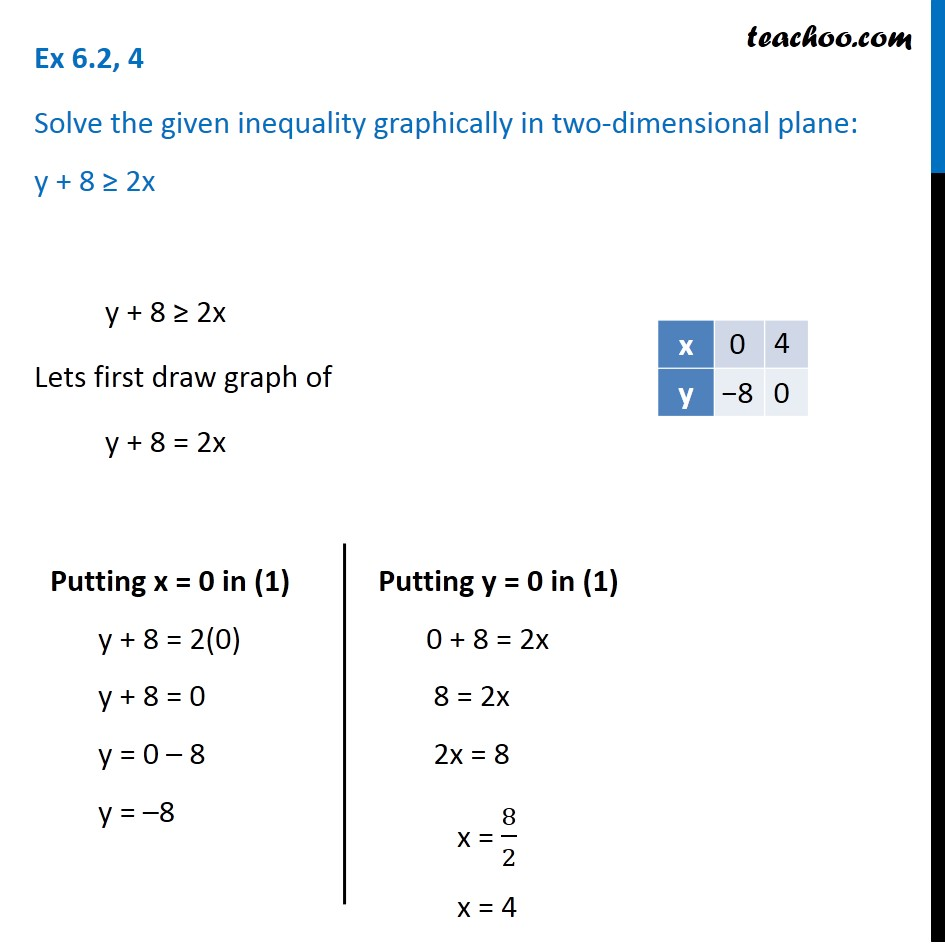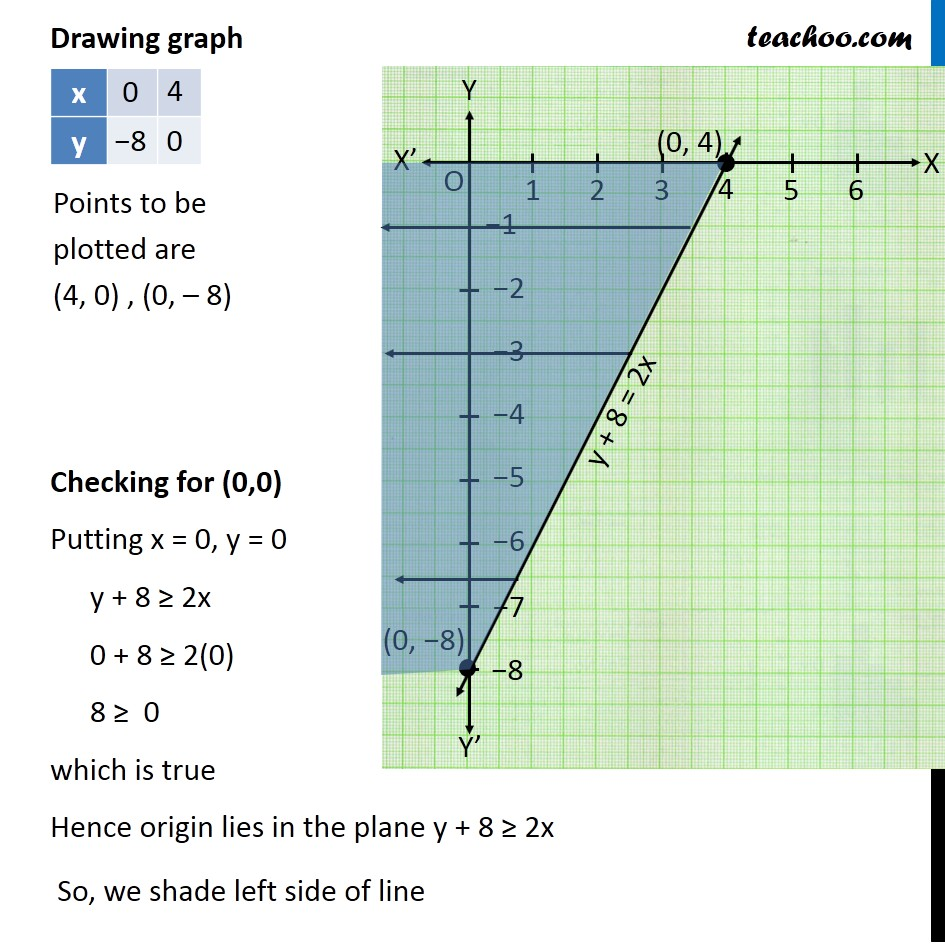Solving Linear Inequality Graphically

Chapter 5 Class 11 Linear Inequalities
Serial order wiseLearn in your speed, with individual attention - Teachoo Maths 1-on-1 Class

### Transcript

Question 4 Solve the given inequality graphically in two-dimensional plane: y + 8 ≥ 2x y + 8 ≥ 2x Lets first draw graph of y + 8 = 2x VPutting y = 0 in (1) 0 + 8 = 2x 8 = 2x 2x = 8 x = 8/2 x = 4 Drawing graph Checking for (0,0) Putting x = 0, y = 0 y + 8 ≥ 2x 0 + 8 ≥ 2(0) 8 ≥ 0 which is true Hence origin lies in the plane y + 8 ≥ 2x So, we shade left side of line Points to be plotted are (4, 0) , (0, – 8)